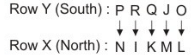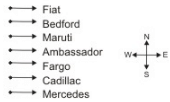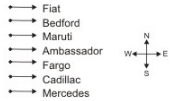# NABARD Manager Reasoning Mock Test 2

## 20 Questions MCQ Test NABARD Manager - Mock Tests & Previous Year Papers | NABARD Manager Reasoning Mock Test 2

Description
Attempt NABARD Manager Reasoning Mock Test 2 | 20 questions in 12 minutes | Mock test for Banking Exams preparation | Free important questions MCQ to study NABARD Manager - Mock Tests & Previous Year Papers for Banking Exams Exam | Download free PDF with solutions
QUESTION: 1

Solution:
QUESTION: 2

### Below are the statements followed by four conclusions numbered I,II,III and IV. You have to consider the statements and the following conclusions and decide which of the conclusion(s) follows the statement(s). Statements : a. Some spectacles are boxes. b. No bat is a ball. c. Some boxes are balls. Conclusions : I. Some boxes are not bats. II. Some bats are spectacles. III. No bat is a box. IV. No ball is a spectacle.

Solution:
Only conclusion I follows
QUESTION: 3

### In the following questions, the symbols @, \$, %, # and * are used with the following meaning as illustrated below: 'P @ Q' means 'P is not smaller than Q'. 'P \$ Q' means 'P is not greater than Q'. 'P % Q' means 'P is neither greater than nor smaller than Q'. 'P # Q' means 'P is neither smaller than nor equal to Q'. 'P * Q' means 'P is neither greater than nor equal to Q'. Now in each of the following questions assuming the given statements to be true, find which of the two conclusions I and II given below them is/are definitely true. Statements : T @ V, V # M, M % F Conclusions : I. T # M II. T @ F

Solution:

T ≥ V > M = F
Conclusions:
I) T > M ; True
II) T ≥ F ; False
∵ T > F and never equal to T
Hence, only conclusion (I) followes

QUESTION: 4

In the following questions, the symbols @, \$, %, # and * are used with the following meaning as illustrated below:
'P @ Q' means 'P is not smaller than Q'.
'P \$ Q' means 'P is not greater than Q'.
'P % Q' means 'P is neither greater than nor smaller than Q'.
'P # Q' means 'P is neither smaller than nor equal to Q'.
'P * Q' means 'P is neither greater than nor equal to Q'.
Now in each of the following questions assuming the given statements to be true, find which of the two conclusions I and II given below them is/are definitely true.

Statements :
L \$ N, N * F, R % L
Conclusions :
I. F # R
II. R \$ N

Solution:

R = L ≤ N < F
Conclusions:
I) F > R ; True
II) R ≤ N ; True
Both the conclusions are true

QUESTION: 5

In the following questions, the symbols @, \$, %, # and * are used with the following meaning as illustrated below:
'P @ Q' means 'P is not smaller than Q'.
'P \$ Q' means 'P is not greater than Q'.
'P % Q' means 'P is neither greater than nor smaller than Q'.
'P # Q' means 'P is neither smaller than nor equal to Q'.
'P * Q' means 'P is neither greater than nor equal to Q'.
Now in each of the following questions assuming the given statements to be true, find which of the two conclusions I and II given below them is/are definitely true.

Statements :
H # I, I @ J, J \$ P
Conclusions :
I. H # J
II. H # P

Solution:

H > I ≥ J ≤ P
Conclusions:
I) H > J ; True
II) H > P ; No relationship
Only conclusion (I) is true

QUESTION: 6

Ten members I, J, K, L, M, N, O, P, Q and R are split into two teams X and Y of 5 members each. Members in the two teams are made to sit in two rows facing each other in such a way that one member of team X is sitting exactly opposite of a member in team Y. Members of team X are facing North. L is third to the right of I and sits exactly opposite of O. J sits to the immediate right of O and is facing South. P is third to the right of J and is exactly opposite of N. K sits between I and M and is opposite of Q.

Q. Who is second to the left of K?

Solution:QUESTION: 7

Ten members I, J, K, L, M, N, O, P, Q and R are split into two teams X and Y of 5 members each. Members in the two teams are made to sit in two rows facing each other in such a way that one member of team X is sitting exactly opposite of a member in team Y. Members of team X are facing North. L is third to the right of I and sits exactly opposite of O. J sits to the immediate right of O and is facing South. P is third to the right of J and is exactly opposite of N. K sits between I and M and is opposite of Q.

Q. Which of the following group of persons sit in the same row?

Solution:QUESTION: 8

Ten members I, J, K, L, M, N, O, P, Q and R are split into two teams X and Y of 5 members each. Members in the two teams are made to sit in two rows facing each other in such a way that one member of team X is sitting exactly opposite of a member in team Y. Members of team X are facing North. L is third to the right of I and sits exactly opposite of O. J sits to the immediate right of O and is facing South. P is third to the right of J and is exactly opposite of N. K sits between I and M and is opposite of Q.

Q. Who is the immediate right of J?

Solution:QUESTION: 9

Each question below consists of two statements numbered I and II . You have to decide whether the data provided in the statements are sufficient to answer the questions.
Give answer (1) if the statement I alone is sufficient to answer the question, but the statement II alone is not sufficient.
Give answer (2) if the statement II alone is sufficient to answer the question, but the statement I alone is not sufficient.
Give answer (3) if both statements I and II together are needed to answer the question.
Give answer (4) if either the statement I alone or statement II alone is sufficient to answer the question.
Give answer (5) if you cannot get the answer from the statement I and II together, but need even more data.

Q. Is x+y-z+t even?
I. x+y+t is even.
II. t and z are odd.

Solution:
QUESTION: 10

Each question below consists of two statements numbered I and II . You have to decide whether the data provided in the statements are sufficient to answer the questions.
Give answer (1) if the statement I alone is sufficient to answer the question, but the statement II alone is not sufficient.
Give answer (2) if the statement II alone is sufficient to answer the question, but the statement I alone is not sufficient.
Give answer (3) if both statements I and II together are needed to answer the question.
Give answer (4) if either the statement I alone or statement II alone is sufficient to answer the question.
Give answer (5) if you cannot get the answer from the statement I and II together, but need even more data.

Q.  What is the number x?
I. The LCM of x and 18 is 36.
II. The HCF of x and 18 is 2.

Solution:
QUESTION: 11

Each question below consists of two statements numbered I and II . You have to decide whether the data provided in the statements are sufficient to answer the questions.
Give answer (1) if the statement I alone is sufficient to answer the question, but the statement II alone is not sufficient.
Give answer (2) if the statement II alone is sufficient to answer the question, but the statement I alone is not sufficient.
Give answer (3) if both statements I and II together are needed to answer the question.
Give answer (4) if either the statement I alone or statement II alone is sufficient to answer the question.
Give answer (5) if you cannot get the answer from the statement I and II together, but need even more data.

Q. What is the length of rectangle ABCD?
I. Area of the rectangle is 48 square units.
II. Length of the diagonal is 10 units.

Solution:
QUESTION: 12

In an Exhibition seven cars of different companies - Cadillac, Ambassador, Fiat, Maruti, Mercedes, Bedford and Fargo are standing facing to east in the following order :
1. Cadillac is next to right of Fargo.
2. Fargo is fourth to the right of Fiat.
3. Maruti car is between Ambassador and Bedford.
4. Fiat which is third to the left of Ambassador, is at one end.

Q. Which of the cars are on both the sides of cadillac car ?

Solution:Fargo and Mercedes are on both the sides of cadillac car.

QUESTION: 13

In an Exhibition seven cars of different companies - Cadillac, Ambassador, Fiat, Maruti, Mercedes, Bedford and Fargo are standing facing to east in the following order :
1. Cadillac is next to right of Fargo.
2. Fargo is fourth to the right of Fiat.
3. Maruti car is between Ambassador and Bedford.
4. Fiat which is third to the left of Ambassador, is at one end.

Q. Which of the following statement is correct ?

Solution:Therefore, Maruti is next left of Ambassador.

QUESTION: 14

Lakshmi: I know that wildflowers are threatened because of the reduced number of suitable spaces where they can grow and that people should generally leave them where they are growing. This large field, however, is full of plants of one wildflower species, and it would be all right for me to take just one plant, since there are so many.
Malini: There will not be many there in the future if many people act on your principle.

Q. Malini’s criticism proceeds by

Solution:
QUESTION: 15

Lakshmi: I know that wildflowers are threatened because of the reduced number of suitable spaces where they can grow and that people should generally leave them where they are growing. This large field, however, is full of plants of one wildflower species, and it would be all right for me to take just one plant, since there are so many.
Malini: There will not be many there in the future if many people act on your principle.

Q. Which one of the following, if true, is the strongest response Lakshmi can make to counter Malini’s criticism?

Solution:
QUESTION: 16

Study the following information carefully and answer the given question:
A word and number arrangement machine when given an input line of words and numbers rearranges them following a particular rule. The following is an illustration of input and rearrangement.
(All numbers are two-digit numbers.)
Input : pink for 25 72 white jar 12 96
Step I : 96 pink for 25 72 white jar 12
Step II : 96 for pink 25 72 white jar 12
Step III : 96 for 72 pink 25 white jar 12
Step IV : 96 for 72 jar pink 25 white 12
Step V : 96 for 72 jar 25 pink white 12
Step VI : 96 for 72 jar 25 pink 12 white
And Step VI is the last step of the rearrangement.
As per the rules followed in the above steps, find out in each of the following questions the appropriate step for the given input. (All numbers are two-digit numbers.)

Q. Input : 16 power failure 61 53 new cost 27
How many steps will be required to complete the rearrangement?

Solution:
QUESTION: 17

Study the following information carefully and answer the given question:
A word and number arrangement machine when given an input line of words and numbers rearranges them following a particular rule. The following is an illustration of input and rearrangement.
(All numbers are two-digit numbers.)
Input : pink for 25 72 white jar 12 96
Step I : 96 pink for 25 72 white jar 12
Step II : 96 for pink 25 72 white jar 12
Step III : 96 for 72 pink 25 white jar 12
Step IV : 96 for 72 jar pink 25 white 12
Step V : 96 for 72 jar 25 pink white 12
Step VI : 96 for 72 jar 25 pink 12 white
And Step VI is the last step of the rearrangement.
As per the rules followed in the above steps, find out in each of the following questions the appropriate step for the given input. (All numbers are two-digit numbers.)

Q. Step II of an Input : 84 ask quit these 39 12 old 51
Which of the following will definitely be the input?

Solution:
QUESTION: 18

Study the following information carefully and answer the given question:
A word and number arrangement machine when given an input line of words and numbers rearranges them following a particular rule. The following is an illustration of input and rearrangement.
(All numbers are two-digit numbers.)
Input : pink for 25 72 white jar 12 96
Step I : 96 pink for 25 72 white jar 12
Step II : 96 for pink 25 72 white jar 12
Step III : 96 for 72 pink 25 white jar 12
Step IV : 96 for 72 jar pink 25 white 12
Step V : 96 for 72 jar 25 pink white 12
Step VI : 96 for 72 jar 25 pink 12 white
And Step VI is the last step of the rearrangement.
As per the rules followed in the above steps, find out in each of the following questions the appropriate step for the given input. (All numbers are two-digit numbers.)

Q. Step III of an input: 63 bed 58 never go home 46 28
How many more steps will be required to complete the rearrangements?

Solution:
QUESTION: 19

Below are the statements followed by two conclusions numbered I and II. You have to consider the statements and the following conclusions and decide which of the conclusion(s) follows the statement(s).

Statements:
a.All jugs are plates.
b. All plates are cups.
c.All cups are bottles.
Conclusions :
I. Some bottles are jugs.
II. All plates are bottles.

Solution:
QUESTION: 20

Below are the statements followed by two conclusions numbered I and II. You have to consider the statements and the following conclusions and decide which of the conclusion(s) follows the statement(s).

Statements:
a. Some jungles are mirrors.
b. All mirrors are houses.Use Code STAYHOME200 and get INR 200 additional OFF Use Coupon Code